# 用R进行模拟

1.按照p=0.2的二项分布，随机生成一个大小为100的向量。

1.
> x
2.

3.
plot(table(x), main = "frequency")

2. 数字变量 按照高斯分布N（μ=1，σ=1）随机生成一个大小为100的向量。

``> x hist(x, main = "")``

# 模拟数据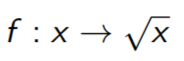1. 定义输入的矢量为x～U[0,1]

2. 定义一个向量u～N+(μ = 0.25, σ = 0.2)

3. 输入的矢量定义为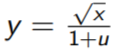1.

2.
> u = rtmvnorm(n = ns, mean = c(0.25), sigma = c(0.2),
3.
+ lower = c(0))
4.
> y = ybar/(1 + u)

# 数据的表示方法

``> plot(y ~ x``

1.

2.
> lines(t.fr ~ x.seq)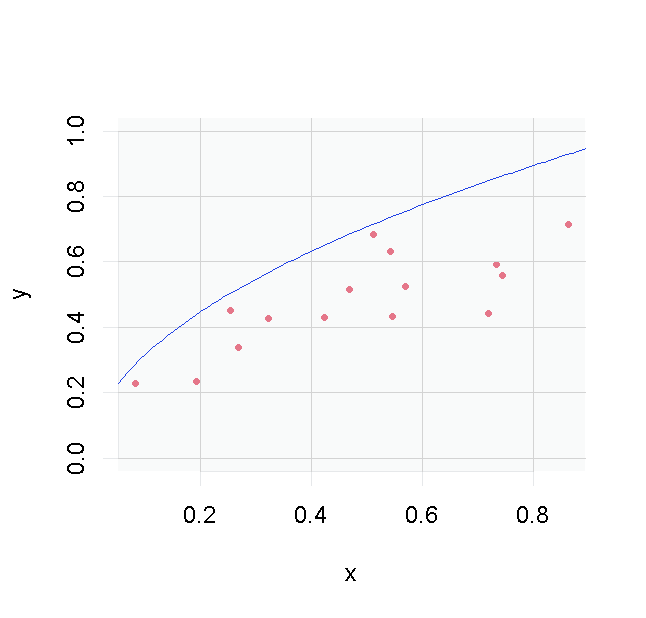# "真实前沿 "效率测算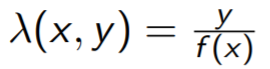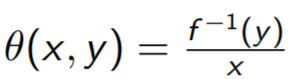Shepard 方法: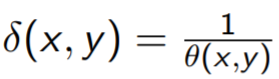1.
> lambda = y/sqrt(x)
2.
> theta = y^2/x
3.
> delta = 1/theta

# 可重复研究

1.

2.
> matable <- xtable(tab1[1:5, ], digits = 3, align = "l|ccc",
3.
+ caption = ""真实前沿效率测算")
4.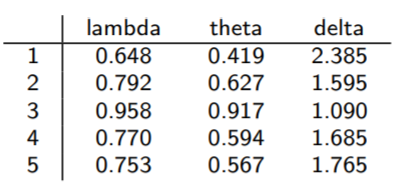# 随机前沿

1.用函数lm调整一个线性模型，并保留回归线的系数β：y = α + βx

2. 找到能使（yi-y?i）最大化的公司k，i=1，...，n。注意，公司k可以通过函数识别来手动找到和检测

3. 计算α 0，使回归线y = α 0 + βx穿过企业k，并代表随机前沿。

## 1. OLS 模型

``````> res.lm <- lm(y ~ x)
``````

## 2. 使用函数识别

1.

2.
> identify(x, y)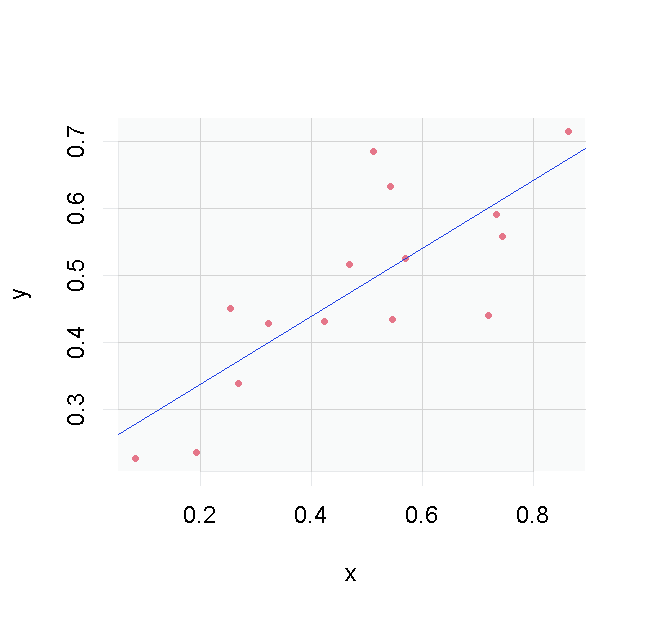## 3. 找到α0

1.
> alpha2 <- y - beta.lm *
2.
+ x
3.
> plot(y ~ x, type = "p",
4.
+ 1))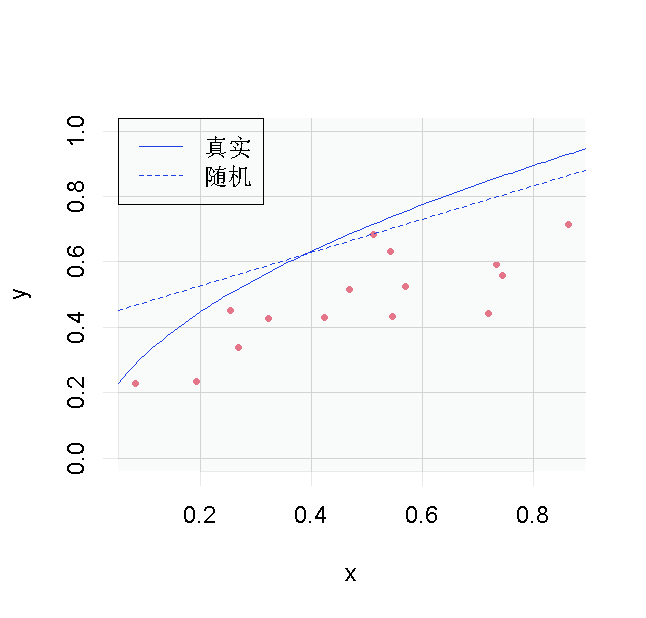# 随机前沿效率测算

``````> f1 = function(x) alpha2 + beta.lm * x
``````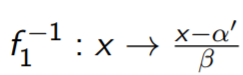``> f1.inv = function(x) (x - alpha2)/beta.lm``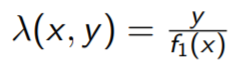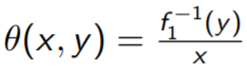Shepard 方法: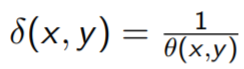# DEA - FDH 表示

1.
> plot(y ~ x)
2.
> lines(x[c(2, 9, 3, 4)], legend = c("DEA",
3.
+ "FDH"))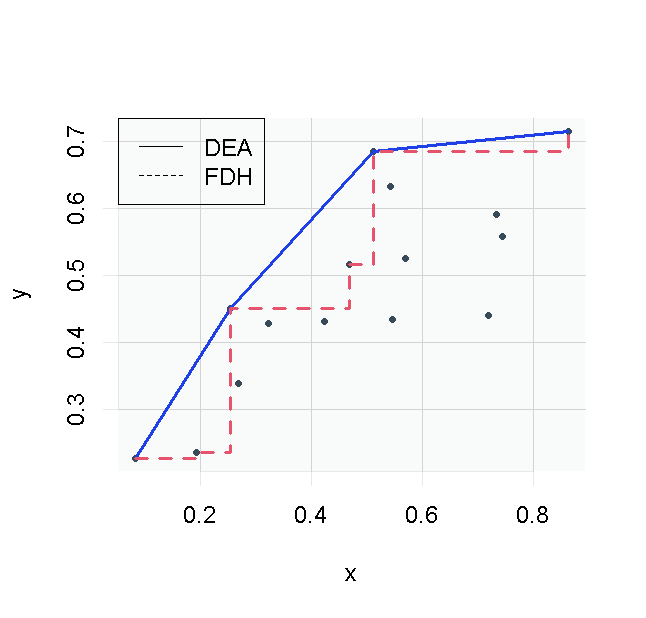# DEA-FDH效率前沿/衡量标准

1. 如果该公司在输出方向上是有效的，它将位于前沿线的哪一部分？ 在输入方向上？

2. 利用这个估计前沿的位置，计算出效率的衡量标准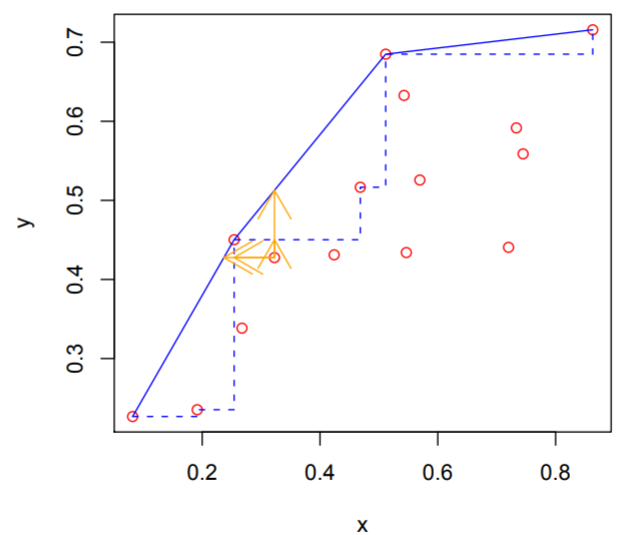# Naive Bootstrap

1.用函数样本在15个观测值中取样

2.计算前沿的新估计值

3.计算新的效率方法

4. 储存结果，计算偏差, 方差, 置信区间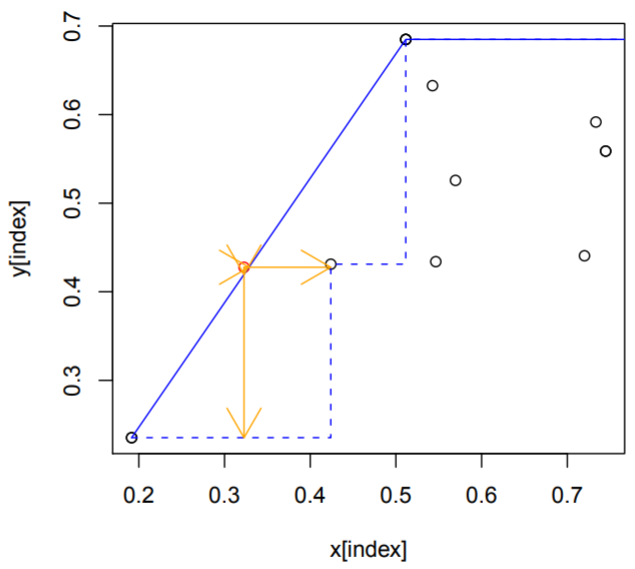# 对真实数据的分析

## 数据集

1.

2.
> plot(y ~ x1,pch = 16, col = "blue")
3.
> abline(lm(y ~ x1, data), col = "red")
4.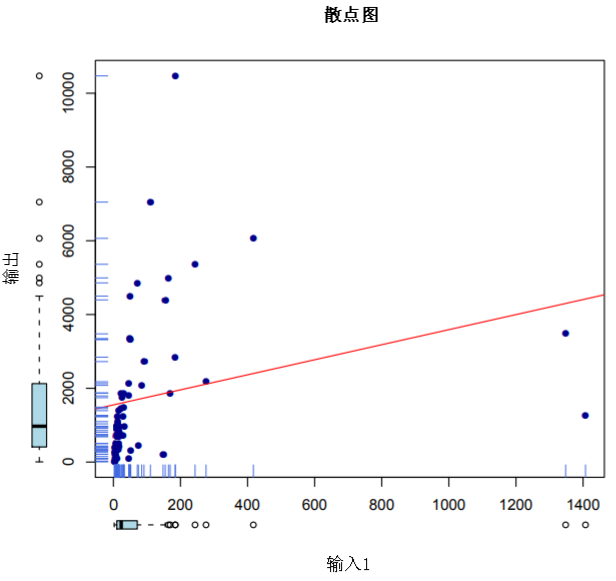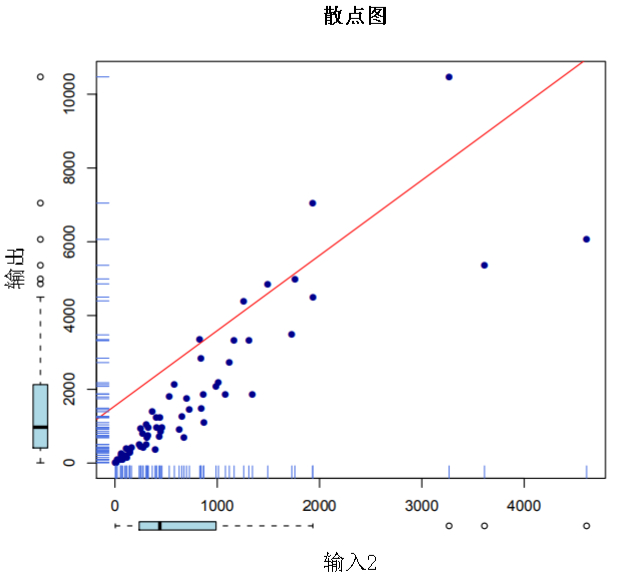# 散点图3-d

1.
> scatterplot3d(x1,
2.
+ x2, y)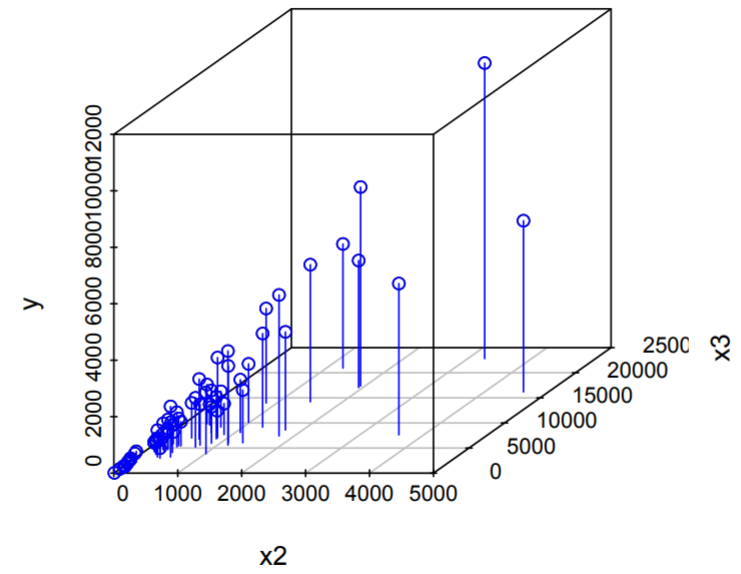# 数据结构

p个输入包含在一个p×n的矩阵中

``````> input <- t(cbind(spain\$x1, spain\$x2, spain\$x3))
``````

q个输出包含在一个q×n的矩阵中

``````> output <- t(matrix(spain\$y))
``````

# 效率的衡量标准

1.

2.
> res <- rbind(dea, fdh, orderm,
3.
+ res.hquan)

``> plot(density(res.dea)``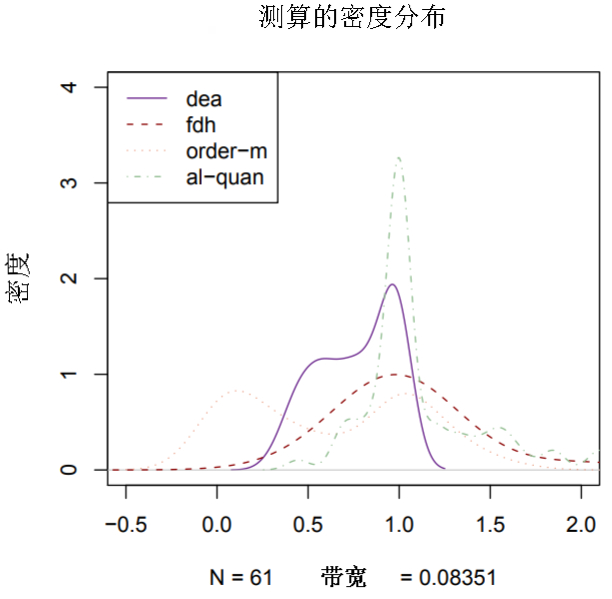# Bootstrap

``````> boot(input, output)
``````(0)
(0)